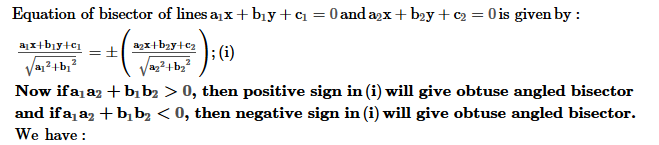# The equation of the line bisecting the obtuse angle between y - x = 2 and 2y + x = 5 is (y-x-2)/(2)1/2 = (x+2y-5)/n. Find n ?

Dear Student ,